# Allez les Bleus !In almost three weeks, the (FIFA) World Cup will start, in Brazil. I have to admit that I am not a big fan of soccer, so I will not talk to much about it. Actually, I wanted to talk about colors, and variations on some colors. For instance, there are a lot of blues. In order to visualize standard blues, let us consider the following figure, inspired by the well known chart of R colors,

BLUES=colors()[grep("blue",colors())]
RGBblues=col2rgb(BLUES)
library(grDevices)
HSVblues=rgb2hsv( RGBblues[1,], RGBblues[2,], RGBblues[3,])
HueOrderBlue=order( HSVblues[1,], HSVblues[2,], HSVblues[3,] )
SetTextContrastColor=function(color) ifelse( mean(col2rgb(color)) > 127, "black", "white")
TextContrastColor=unlist( lapply(BLUES, SetTextContrastColor) )
c=11
l=6
plot(0, type="n", ylab="", xlab="",axes=FALSE, ylim=c(0,11), xlim=c(0,6))
for (j in 1:11){
for (i in 1:6){
k=(j-1)*6 + i
rect(i-1,j-1,i,j, border=NA, col=BLUES[ HueOrderBlue[k] ])
text(i-.5,j-.5,paste(BLUES[k]), cex=0.75, col=TextContrastColor[ HueOrderBlue[k] ])}}

All the color names that contain “blue” in it are here.Having the choice between several possible colors is interesting, but it can also be interesting to get a palette of blue colors, What we can get is the following

library(RColorBrewer)
blues=colorRampPalette(brewer.pal(9,"Blues"))(100)In order to illustrate the use of palette colors, consider some data, on soccer players (officially registered). The dataset – lic-2012-v1.csv – can be downloaded from http://data.gouv.fr/fr/dataset/… (I will also use a dataset we have on location of all towns, in France, with latitudes and longitudes)

base1=read.csv(
"http://freakonometrics.free.fr/popfr19752010.csv",
base1$cp=base1$dep*1000+base1$com base2=read.csv("lic-2012-v1.csv", header=TRUE) base2=base2[base2$fed_2012==111,]
names(base2)="cp"
base2$cp=as.numeric(as.character(base2$cp))

The problem with France (I should probably say one of the many problems) is that regions and departements are not well coded, in the standard functions. To explain where départements are, let us use the dept.rda file, and then, we can get a matching between R names, and standard (administrative) ones,

base21=base2[,c("cp","l_2012","pop_2010")]
base21$dpt=trunc(base21$cp/1000)
library(maps)
base21$nomdpt=dept$dept[match(as.numeric(base21$dpt),dept$CP)]
L=aggregate(base21$l_2012,by=list(Category=base21$nomdpt),FUN=sum)
P=aggregate(base21$pop_2010,by=list(Category=base21$nomdpt),FUN=sum)
base=data.frame(D=P$Category,Y=L$x/P$x,C=trunc(L$x/P$x/.0006)) france=map(database="france") matche=match.map(france,base$D,exact=TRUE)
map(database="france", fill=TRUE,col=blues[base$C[matche]],resolution=0) Here are the rates of soccer players (with respect to the total population)It is also possible to look at rate not by département, but by town, base10=base1[,c("cp","long","lat","pop_2010")] base20=base2[,c("cp","l_2012")] base=merge(base10,base20) Y=base$l_2012/base$pop_2010 QY=as.numeric(cut(Y,c(0,quantile(Y,(1:99)/100),10),labels=1:100)) library(maps) map("france",xlim=c(-1,1),ylim=c(46,48)) points(base$long,base$lat,cex=.4,pch=19,col=blues[QY]) The darker the dot, the more player,We can also zoom in, to get a better understanding, in the northern part of France, for instance,or in the Southern part,We can obtain a map which is not (too) far away from the one mentioned a few months ago on http://slate.fr/france/78502/. # UEFA, is that it ? Following my previous post, a few more things. As mentioned by Frédéric, it is – indeed – possible to compute the probability of all pairs. More precisely, all pairs are not as likely to occur: some teams can play against (almost) eveyone, while others cannot. From the previous table, it is possible to compute probability that the last team plays against team 1. Or team 2 (numbers are from the xls file mentioned previously). To make it simple > table(M[,2*n])/length(M[,2*n])*100 1 2 3 5 7 10 11 11.82500 12.61212 12.61212 13.25279 19.31173 18.70767 11.67856 Here, the last team (as I did rank them) has 11.8% chances to play against team 1, and 19.3% to play against team 7. If we compute all the probabilities, we obtain > S 1 2 3 5 7 10 11 13 4 0.00 14.16 14.16 0.00 22.22 21.25 13.05 15.13 6 12.52 13.19 13.19 14.11 20.13 0.00 12.35 14.47 8 18.78 0.00 19.54 21.50 0.00 0.00 18.39 21.76 9 18.78 19.54 0.00 21.50 0.00 0.00 18.39 21.76 12 14.68 15.54 15.54 16.56 0.00 23.19 14.47 0.00 14 11.64 12.37 12.37 13.05 18.96 18.25 0.00 13.34 15 11.77 12.55 12.55 0.00 19.36 18.59 11.64 13.50 16 11.82 12.61 12.61 13.25 19.31 18.70 11.67 0.00 that can be visualized belowWhite areas cannot be reached, while red ones are more likely. Here, we compute probability that home team (given on the x-axis) plays against some visitor team (on the y-axis). The fact that those probabilities are not uniform seems odd. But I guess it comes from those constraints… Another weird point: it is possible to reach a deadlock. At least with the technique I have been using. So far, I did not count them. But we can, simply the following code > U=c(4,6,8,9,12,14,15,16) > a1=U > b1=U > c1=U > d1=U > e1=U > f1=U > g1=U > h1=U > a2=b2=c2=d2=e2=f2=g2=h2=NA > posa2=(1:n)%notin%c(LISTEIMPOSSIBLE[,a1]) > if(length(posa2)==0){na=na+1} > for(a2 in posa2){ + posb2=(1:n)%notin%c(LISTEIMPOSSIBLE[,b1],a2) + if(length(posb2)==0){na=na+1} + for(b2 in posb2){ + posc2=(1:n)%notin%c(LISTEIMPOSSIBLE[,c1],a2,b2) + if(length(posc2)==0){na=na+1} + for(c2 in posc2){ + posd2=(1:n)%notin%c(LISTEIMPOSSIBLE[,d1], + a2,b2,c2) + if(length(posd2)==0){na=na+1} + for(d2 in posd2){ + pose2=(1:n)%notin%c(LISTEIMPOSSIBLE[,e1], + a2,b2,c2,d2) + if(length(pose2)==0){na=na+1} + for(e2 in pose2){ + posf2=(1:n)%notin%c(LISTEIMPOSSIBLE[,f1], + a2,b2,c2,d2,e2) + if(length(posf2)==0){na=na+1} + for(f2 in posf2){ + posg2=(1:n)%notin%c(LISTEIMPOSSIBLE[,g1], + a2,b2,c2,d2,e2,f2) + if(length(posg2)==0){na=na+1} + for(g2 in posg2){ + posh2=(1:n)%notin%c(LISTEIMPOSSIBLE[,h1], + a2,b2,c2,d2,e2,f2,g2) + if(length(posh2)==0){na=na+1} + for(h2 in posh2){ + s=s+1 + V=c(a1,a2,b1,b2,c1,c2,d1,d2,e1,e2,f1,f2,g1,g2,h1,h2) + }}}}}}}} On the initial ordering of home team, the number of deadlocks was > na  657 The probability of obtaining a deadlock is then > 657/(657+5463)  0.1073529 (657 scenarios ended in a dead end, while 5463 ended well). The worst case was obtained when we considered   6 4 16 14 12 15 8 9 In that case, the probability of obtaining a deadlock was > 4047/(4047+5463)  0.4255521 Here, it clearly depends on the ordering. So if we draw – randomly – the order of the home teams, i.e. > Urandom=sample(U,size=8) the distribution of the probablity of having a deadlock isAll those computations were based on my understanding of the drawings. But Kristof (aka @ciebiera), on his blog krzysztofciebiera.blogspot.ca/… obtained different results. For instance, based on my previous computations, the probability to obtain identical pairs was 0.018349% (1 chance out of 5463), but Kristof obtained – based on the UEFA procedure (as he called it) – a probability of 0.0181337%. Which is not _ strictly – the same, but both computations yield relatively close results… # UEFA, what were the odds ? Ok, I was supposed to take a break, but Frédéric, professor in Tours, came back to me this morning with a tickling question. He asked me what were the odds that the Champions League draw produces exactly the same pairings from the practice draw, and the official one (see e.g. dailymail.co.uk/…). To be honest, I don’t know much about soccer, so here is what happened, with the practice draw (on the left, on December 19th) and the official one (on the right, on December 20th),Clearly, the pairs are identical, but not the order. Actually, at first, I was suprised that even which team plays at home first, was iddentical. But (it seams that) teams that play at home first are the ones that ended second after the previous stage of the competition. And to be more specific about those draws, those pairs were obtained using real urns, real balls, so it is pure randomness (again, as far as I understood). But with very specific rules. For instance, two teams from the same country cannot play together (or one against the other) at this stage. Or teams that ended first after the previous turn can only play with (or against) teams that ended second. Actually, Frederic sent me an xls file, with a possibility matrix. Let us find all possible pairs, regardless which team plays at home first (again, we do not care here since the order is defined by the rule mentioned above). Doing the maths might have been a bit complicated, with all those contraints. With a small code, it is possible to list all possible pairs, for those eight games. Let us import our possibility matrix,  > n=16 > uefa=read.table( + "http://freakonometrics.blog.free.fr/public/data/uefa.csv", + sep=",",header=TRUE) > LISTEIMPOSSIBLE=matrix( + (rep(1:n,n))*(uefa[1:n,2:(n+1)]=="NON"),n,n) I can fix the first team (in my list, the fourth one is the first team that ended second). Then, I look at all possible second one (that will play with the first one),  > a1=1 > "%notin%" <- function(x, table){x[match(x, table, nomatch = 0) == 0]} > posa2=((a1+1):n)%notin%LISTEIMPOSSIBLE[,a1] Then, consider the second team that ended second (the sixth one in my list). And look at all possible fourth team (that will play this second game), i.e exluding the one that were already drawn, and those that are not possible,  > b1=6 > posb2=(1:n)%notin%c(LISTEIMPOSSIBLE[,b1],a2) Etc. So, given the list of home teams,  > a1=4 > b1=6 > c1=8 > d1=9 > e1=12 > f1=14 > g1=15 > h1=16 consider the following loops,  > posa2=(1:n)%notin%c(LISTEIMPOSSIBLE[,a1]) > for(a2 in posa2){ + posb2=(1:n)%notin%c(LISTEIMPOSSIBLE[,b1],a2) + for(b2 in posb2){ + posc2=(1:n)%notin%c(LISTEIMPOSSIBLE[,c1],a2,b2) + for(c2 in posc2){ + posd2=(1:n)%notin%c(LISTEIMPOSSIBLE[,d1],a2,b2,c2) + for(d2 in posd2){ + pose2=(1:n)%notin%c(LISTEIMPOSSIBLE[,e1],a2,b2,c2,d2) + for(e2 in pose2){ + posf2=(1:n)%notin%c(LISTEIMPOSSIBLE[,f1],a2,b2,c2,d2,e2) + for(f2 in posf2){ + posg2=(1:n)%notin%c(LISTEIMPOSSIBLE[,g1],a2,b2,c2,d2,e2,f2) + for(g2 in posg2){ + posh2=(1:n)%notin%c(LISTEIMPOSSIBLE[,h1],a2,b2,c2,d2,e2,f2,g2) + for(h2 in posh2){ + s=s+1 + V=c(a1,a2,b1,b2,c1,c2,d1,d2,e1,e2,f1,f2,g1,g2,h1,h2) + cat(s,V,"\n") + M=rbind(M,V) + }}}}}}}} With the print option, we end up with 5461 4 13 6 11 8 5 9 2 12 10 14 3 15 7 16 1 5462 4 13 6 11 8 5 9 2 12 10 14 7 15 1 16 3 5463 4 13 6 11 8 5 9 2 12 10 14 7 15 3 16 1 i.e. > nrow(M)  5463 possible pairs (the list can be found here, where numbers are the same as the one in the csv file). Which was the probability mentioned in acomment in the article mentioned previously dailymail.co.uk/…. So the probability to have exactly the same output after the practise and the official draws was (in %) > 100/nrow(M)  0.01830496 Which is not that small when we think about it…. And if someone has a mathematical expression for this probability, I am interested. The only reliable method I found was to list all possible pairs (the csv file is available if someone wants to check). But I am not satisfied…. # STT2700, estimation, tests et coupes du mondeMercredi, pour le dernier cours, nous allons revenir sur l’estimation, les tests, et plus généralement sur la modélisation statistique. Pour cela, j’avais pensé travailler sur les nombres de buts marqués, par match, lors de différentes coupes du monde de soccer (1982, 1998 et 2010). Je ne mets pas l’intégralité du code aujourd’hui, l’idée est pour l’instant de mettre en ligne des données qui serviront à répondre aux questions qui seront posées mercredi. Le code (accompagné – éventuellement – d’explications théoriques) sera posté par la suite. soccer1982=read.table("http://freakonometrics.free.fr/soccer1982") S82=(soccer1982$V1+soccer1982$V2) soccer1998=read.table("http://freakonometrics.free.fr/soccer1998") S98=(soccer1998$V1+soccer1998$V2) soccer2010=read.table("http://freakonometrics.free.fr/soccer2010") S10=(soccer2010$V1+soccer2010\$V3)

Les boxplot associés à ces trois échantillons sont les suivants,On va se poser des questions autour de ces données, par exemple voir s’il est vraisemblance (ou pas) que le nombre moyen de but dans un match (avant prolongation, s’il y en a eu). On peut commencé par essayer de se demander quel modèle utiliser. Classiquement, la loi de Poisson est la plus utilisée (en plus, c’est la seule loi qui est autorisée lorsqu’on publie un billet le 1er avril). Les histogrammes sont les suivants

boxplot(S82,S98,S10,col=c("red","yellow","blue"),
label=("1982","1998","2010"))
hist(S82,breaks=0:11,col="red")
hist(S98,breaks=0:11,col="yellow")
hist(S10,breaks=0:11,col="blue")Si on compare les fonctions de répartition empiriques à celles de lois de Poisson ajustées par maximum de vraisemblance, on obtient, pour la coupe du monde de 1982et pour celle de 2010,Visuellement, l’ajustement semble relativement bon, surtout en 2010. On peut aussi faire un test du chi-deux,

> library(vcd)
> (GF=goodfit(S10,type="poisson"))

Observed and fitted values for poisson distribution
with parameters estimated by ML

count observed     fitted
0        7  6.6409703
1       17 15.0459484
2       13 17.0442384
3       14 12.8719508
4        7  7.2907534
5        5  3.3036226
6        0  1.2474617
7        1  0.4037543

> summary(GF)

Goodness-of-fit test for poisson distribution

X^2 df  P(> X^2)
Likelihood Ratio 5.586765  5 0.3485255

On voit que l’on accepte l’ajustement par une loi de Poisson. Pour ceux qui veulent une visualisation, sur la figure ci-dessous, on a la densité d’une loi du chi-deux. Le premier trait vertical est la valeur observée, et l’aire jaune est alors la p-value (qui excède largement 5%). En rouge on a 5%, donc le second trait vertical est la borne de la région critique associé au test pour une erreur de première espèce valant 5%,On peut ensuite faire plein de tests.  On suppose que . On va pouvoir tester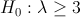contre une hypothèse alternative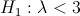Comme on a une hypothèse sur la loi des observations qui semble robuste, on peut utiliser un test de type rapport de vraisemblance.
On peut aussi faire un test de la forme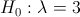contre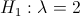(histoire de tester des hypothèses simples – qui ont une interprétation). Sinon, comme ce qui nous intéresse, c’est de savoir si on a plus de trois buts par matchs, on peut définir la variable binomiale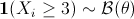, en notant que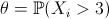est une proportion – donc facile à tester – qui nous intéresse ici compte tenu du problème que l’on cherchera à résoudre. On pourra alors tester, par exemple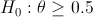contre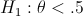Ces derniers tests sont alors facile à mettre en œuvre,

> Z=(S10>=3)*1
> prop.test(sum(Z),length(Z),p=1/2,alternative="less")

1-sample proportions test with continuity correction

data:  sum(Z) out of length(Z), null probability 1/2
X-squared = 1.2656, df = 1, p-value = 0.1303
alternative hypothesis: true p is less than 0.5
95 percent confidence interval:
0.0000000 0.5322764
sample estimates:
p
0.421875

On peut aussi faire des tests de moyenne sur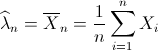Un test de l’hypothèsecontre une hypothèse alternatives’écrit alors
> t.test(S10,mu=3,alternative ="less")

One Sample t-test

data:  S10
t = -3.7763, df = 63, p-value = 0.0001775
alternative hypothesis: true mean is less than 3
95 percent confidence interval:
-Inf 2.590273
sample estimates:
mean of x
2.265625
Mais on l’aura l’occasion de revoir tous les points du cours, y compris aller peut être un peu plus loin, par exemple sur la comparaison de moyenne entre échantillons,
> t.test(S82,S98,var.equal=FALSE)

Welch Two Sample t-test

data:  S82 and S98
t = 0.427, df = 85.266, p-value = 0.6704
alternative hypothesis: true difference in means is not equal to 0
95 percent confidence interval:
-0.5503669  0.8514658
sample estimates:
mean of x mean of y
2.807692  2.657143

# L’équipe de France de foot devrait jouer seulement en semaine (ça sauverait des vies)

Il y a quelques semaines, le service de centralisation des statistiques de l’assurance accidents LAA, par l’intermédiaire de Stefan Scholz Odermatt, a publié une étude sur la survenance d’accident automobile les jours de match de foot.Selon l’étude (mentionnée ici ou ) le nombre d’accidents les jours où il y a match est supérieur aux jours sans match,Ayant réussi à récupérer une base presque exhaustive d’accidents corporels en France, je peux faire la même étude, entre janvier 2002 et décembre 2007. Les conclusions sont moins flagrantes que sur le cas suisse, en particulier les jours de semaine, où manifestement, personne ne s’intéresse aux matchs (où en tous les cas pas assez pour être moins vigilent au volant).En revanche, le week end, l’effet devient plus prononcéavec davantage d’accidents dans la journée où il y a un match de l’équipe de France.

Moralité, l’équipe de France de foot devrait jouer seulement en semaine, à chaque match, cela éviterait une vingtaine d’accidents de la route à chaque fois (et je ne parle que d’accidents corporels, ayant causé des blessures et ayant donné lieu à un constat de police) ! Comme le disait un copain à qui je racontais ça, “l’équipe de France devrait jouer, ça serait un début“…. mais c’est un autre sujet.